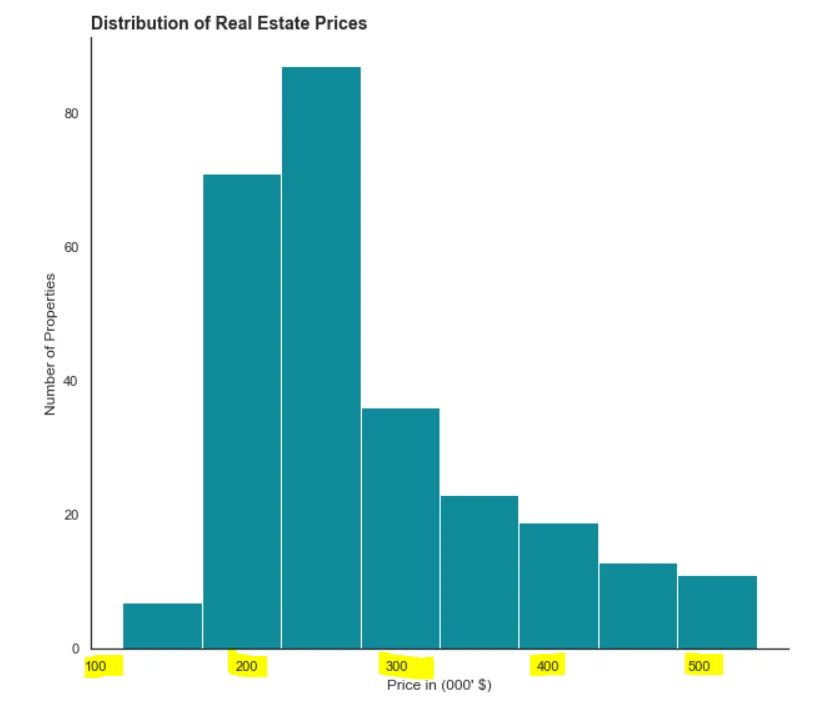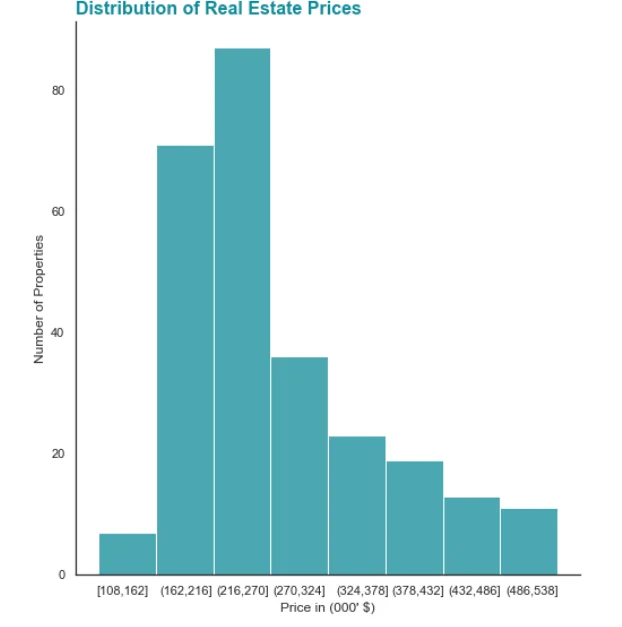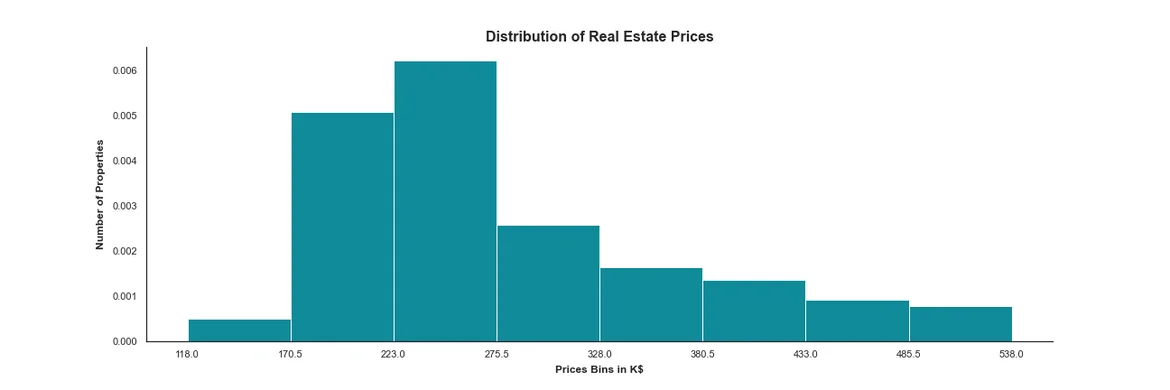20 Nov 2022

Posted on:

06 Jul 2022

0

# Editing the X axis in matplotlib

How can I add a better X axis on my histogram using matplotlib? Is there a way to add the notation (x,y]?

``````sns.set_style("white")
plt.figure(figsize = ( 10,9))
plt.hist(df_real_estate["Price"],
bins = 8,
color = "#108A99")
plt.title("Distribution of Real Estate Prices", loc = "left", fontweight = "bold", fontsize = 14)
plt.ylabel("Number of Properties")
plt.xlabel("Price in (000' \$)")
sns.despine()
plt.show()
``````Posted on:

06 Jul 2022

0

I was able to hard code the labels with sns.histplot (set_xticks,set_xticklabels) ...but I would have to do this if the number of bins changes. Is there a better solution? I looked online without much success. Thank you

``````sns.set_style("white")
plt.figure(figsize = (8,9))
h = sns.histplot(df_real_estate["Price"], bins =8, color = "#108A99")
h.set_xticks([140,198,250,302,360,410,462,515])
h.set_xticklabels(["[108,162]","(162,216]","(216,270]","(270,324]","(324,378]","(378,432]","(432,486]","(486,538]"])
plt.title("Distribution of Real Estate Prices", loc = "left", fontweight = "bold", fontsize = 16, color = "#108A99")
plt.ylabel("Number of Properties")
plt.xlabel("Price in (000' \$)")
sns.despine()
plt.show()
``````Posted on:

20 Nov 2022

0

I think a quick solution is by indicating the start and the end of each interval within plt.xticks function
this can be done by importing numpy library and using arange function (a function that returns a list of values based on three parameters; start, step and stop values
also we shall use np.ptp function which calculates the difference between min and max of an array)

``````import numpy as np
bins=8 #this a variable defining the number of bins, you can change it to any number you want
plt.xticks(ticks=np.arange(start=min(df_hist["Price"]),
step=np.ptp(df_hist["Price"])/bins,
stop=np.max(df_hist["Price"])+1))
``````

the resulting graph should be as follow taking into consideration that the start of each interval is not included is not included in the bar except for the first oneto get the labels to be graphed the same way as in excel (square and round brackets used), you should create a loop or to indicate them manually in labels parameter in plt.xticks